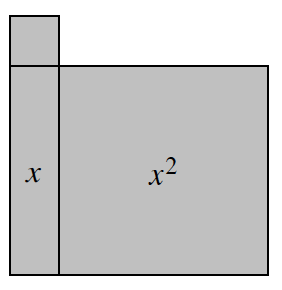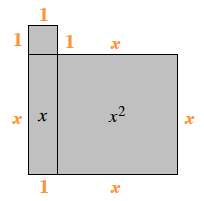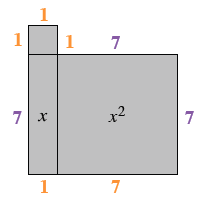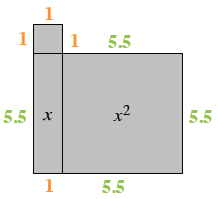### Home > CCAA > Chapter 6 Unit 4 > Lesson CC2: 6.1.4 > Problem6-45

6-45.

On your paper, sketch the algebra tile shape at right. Write an expression for the perimeter, and then find the perimeter for each of the given values of $x$.The perimeter is the sum of all the sides. Mark each side with its length to help you find the perimeter. The same algebra tile digram, as the problem, with the following lengths, starting on the top left, and going clockwise: 1, 1, x, x, x, 1, x, 1.

Combine like terms to form an expression of the perimeter.1. $x=7$ cm

Substitute $7$ for $x$ in the algebra tile shape or the expression you found to find the perimeter for this given value.  The same algebra tile digram, with the following lengths, starting on the top left, and going clockwise:1, 1, 7, 7, 7, 1, 7, 1.

1. $x=5.5$ cm

Substitute $5.5$ for $x$ in the algebra tile shape or the expression you found to find the perimeter for this given value.  The same algebra tile digram, as the problem, with the following lengths, starting on the top left, and going clockwise: 1, 1, 5.5, 5.5, 5.5, 1, 5.5, 1.